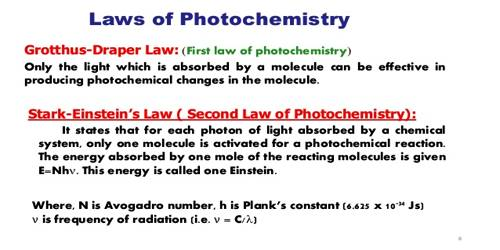# The Laws of Photochemistry

The Laws of Photochemistry

There are two basic laws of photochemistry. In 1817 Grotthus and later Draper stated that only that radiation which is absorbed by a substance is effective in producing a photochemical reaction. This is known as the Grotthus-Draper law. It does not follow, however, that all the light that is absorbed will produce a reaction.

A significant advance was made by Einstein and later by Stark who applied the concept of the quantum of energy to photochemical reactions of molecules. They formulated a law, known as the law of photochemical equivalence, which states that – each molecule taking pan in a reaction induced by exposure to radiant energy absorbs one quantum of radiation causing the reaction. It should be emphasized that this applies only to the primary process of light absorption. A molecule on the absorption of energy does not necessarily undergo reaction; again one activated molecule may cause the reaction of a large number of molecules through a chain mechanism.

The First Law of Photochemistry states that light must be absorbed for photochemistry to occur. This is a simple concept, but it is the foundation for performing photochemical and photobiological experiments correctly. This law also is known as the Grotthuss-Draper law, states that light must be absorbed by a compound in order for a photochemical effect to take place.

The Second Law of Photochemistry states that for each photon of light absorbed by a chemical system, only one molecule is activated for a photochemical reaction. This law also is known as, the Stark-Einstein law, states that for each photon of light absorbed by a chemical system, only one molecule is activated for a consequent reaction.Hence the law should not be taken as to mean that one molecule reacts for each quantum absorbed. The ratio of the number of molecules reacted to the number of quanta of radiation absorbed is called the quantum efficiency or quantum yield (Φ). i.e.,

Φ = [Number of molecules reacted / Number of quanta absorbed]

This then is the relation between the amount which undergoes photochemical reaction and the amount of radiant energy absorbed. In an idealized system, the quantum yield should be unity. For several reactions, Φ = 1, for many reactions Φ ˃ 1, while for other Φ < 1.

As a matter of fact, on one from the law of photochemical equivalence arc considered to be due to one or more side-effects and explanations for these have been offered. In some cases, quantum yield of 2 is in accordance with the Einstein-Stark law. For example, the photochemical reaction between hydrogen and iodine takes place according to the following scheme:

Each quantum of absorbed radiation forms two hydrogen iodide molecules, yielding a Φ value of 2. However, in the reaction between hydrogen and chlorine θ is very large and may assume a value of 106. Such high values of θ definitely indicate a chain mechanism in the reaction. Several reactions are also known where θ assumes values much lower than unity. These low values are mainly due to fluorescence, phosphorescence and other side-effects. When a solution of anthracene in benzene is exposed to ultraviolet radiation, two molecules of anthracene combine to form anthracene, 2C14H10 → C28H20. The reaction is accompanied by fluorescence and the quantum yield is low in dilute solution due to de-excitation. When the concentration of anthracene is increased in the solution the quantum yield increases whereas fluorescence decreases. At sufficiently high concentration the quantum yield assumes a limiting constant value and fluorescence practically disappears.